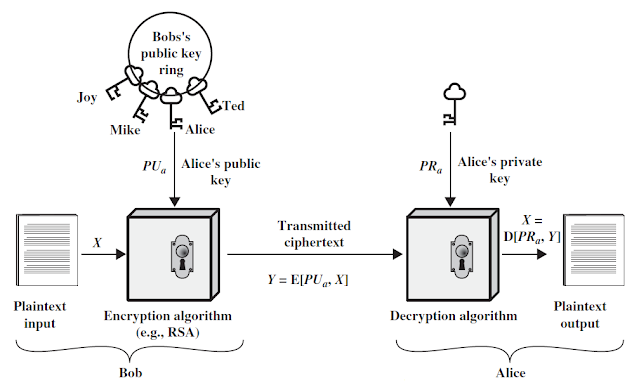## Tuesday, September 14, 2021

### Asymmetric Key Cryptography | Public Key Cryptography

Asymmetric Key Cryptography (Public Key Cryptography)

Different keys are used for encryption and decryption, it is called asymmetric key cryptography. Public key used for encryption, private key used for decryption and vice versa. It is also known as public key cryptography.

Elements of Asymmetric Key Cryptography

Plaintext is the original message or data that is fed into the algorithm as input.

Encryption algorithm performs various substitutions and transformations on the plaintext.

Public & Private keys: This is a pair of keys that have been selected so that if one is used for encryption, the other is used for decryption.

Ciphertext is the unreadable message produced as output. It depends on the plain text and secret key.

Decryption algorithm takes the cipher text and the secret key and produces the original plain text.

Public key is distributed to all users and Private key is known to particular user only.

There are two different Scenario of encryption model:

Public key used for encryption and private key used for decryption.

Private key used for encryption and public key used for decryption.

Scenario – 1: Encryption with public key

If Bob wants to send message to Alice, Bob must have to use public key of Alice. Message to be transmitted after encryption of message using Alice’s public key.

Alice has received message and she can decrypt the message using only her private key’s.Figure: Encryption with Public Key

Mathematically, it is represented,

Y = E(Pu(A), X)

X = D(Pr(A), Y).

Where, Pu(A) = Alice public key and Pr(A) = Alice private key.

Scenario – 2: Encryption with private key

If Bob wants to send message to Alice, Bob must have to use his own private key. Message to be transmitted after encryption of message using Bob’s public key.

Alice has received message and she can decrypt the message using Bob’s public key.Figure: Encryption with Private Key

Mathematically, it is represented,

Y = E(Pr(B), X)

X = D(Pu(B), Y).

Where, Pr(B) = Bob’s private key, Pu(B) = Bob’s public key.